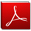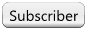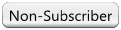# International Journal of Aerospace and Lightweight Structures (IJALS)

Volume 3 Number 1 (2013)

International Journal of Aerospace and Lightweight Structures

doi: 10.3850/S201042862013000574

# Multiphase Trajectory Optimization for Hypersonic Vehicle Using Gauss Pseudospectral Method

Yuyang Shi1,a, Zi-ming Wan2 and Min Xu1
1Department of Astronautics, Northwestern Polytechnical University, Xi’an, 710072, China.
asunrainny@163.com
2The Second System Design Department of the Second Research Academy of CASIC, Beijing, 100854, China.

## ABSTRACT

This paper shows the application of the Gauss pseudospectral method (GPM) to solve a multiple phase maximum range trajectory optimization problems for hypersonic vehicles in the presence of constraints such as dynamic pressure and heat flux. Unlike most trajectory optimization problems, the path of hypersonic vehicle has to undergo large change in altitude and associated aerodynamic conditions. The trajectory optimization of hypersonic vehicle is a nonlinear, path constrained and terminal constraint discontinuous optimal control problem. The Gauss pseudospectral method is based on using global Lagrange interpolating polynomial approximation to the dynamic equation at a set of Gauss collocation points, it has been shown to be accurate numerical methods and possesses fast convergence characteristic for solving optimal control problems. To solving multiphase optimal control problem, a piecewise pseudospectral method is proposed. Firstly, dynamic equation and optimization model are described. Then, control variables and state variables are parameterized, and trajectory optimization problem is transcribed into a mathematical programming problem using the Gauss pseudospectral method. The resulting parameter optimization problem is solved by Sequential Quadratic Program- ming algorithms. To verify the precision of optimal solution, dynamic equations are solved with a fourth-order Runge-Kutta method, which angle of attack obtained from the optimization. The simulation results show that the Gauss pseudospectral method is appropriate for solving multiple-phase trajectory optimization problem, and the method is not sensitive to initial guess, have a high accuracy solution and converge faster.

Keywords: Dynamic equation, Gauss pseudospectral, Hypersonic vehicle, Trajectory optimization.

Back to TOC

FULL TEXT(PDF)